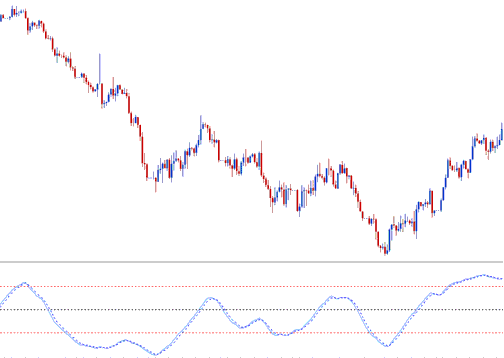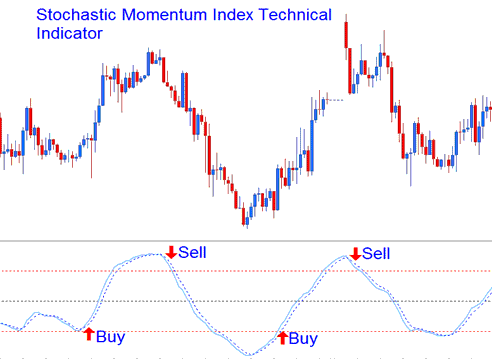# Stochastic Momentum Index Gold Trading Analysis and Stochastic Momentum Index Gold Trading Signals

Developed by William Blau.

The Stochastic Momentum Index, SMI is an adaptation of the classic Stochastic Oscillator that smoothes out the stochastic oscillations.## Construction of Stochastic Momentum Index

This indicator is calculated by comparing the xauusd price relative to the average of an n number of periods.

Then instead of plotting these values directly, smoothing using an Exponential Moving Average is applied and then the values drawn to form the SMI.

When the closing xauusd price is greater than the average of the range, the SMI will move up.

When the closing xauusd price is less than the average of the range, the SMI will move down.

This oscillator ranges between the values of +100 and -100, this indicator is also less prone to whipsaws compared to the stochastic oscillator.

The Stochastic Momentum Index can be used to generate buy and sell xauusd signals using the method shown below, Buy when the SMI is heading upwards and sell when its heading downwards.## Overbought/Oversold Level Crossovers

• Overbought levels above +40

• Oversold levels below -40

Buy signal is generated when this oscillator falls below oversold level and then rises above this level and starts to move upwards.

Sell Gold Trading Signal is generated when this oscillator rises above overbought level and then falls below this level and starts to move downwards.

The example illustrated and explained below shows a bearish classic divergence between the xauusd price and the SMI. When the Stochastic Momentum Index showed this divergence the xauusd price gold trend reversed and started to move in a downward direction.Bearish Divergence